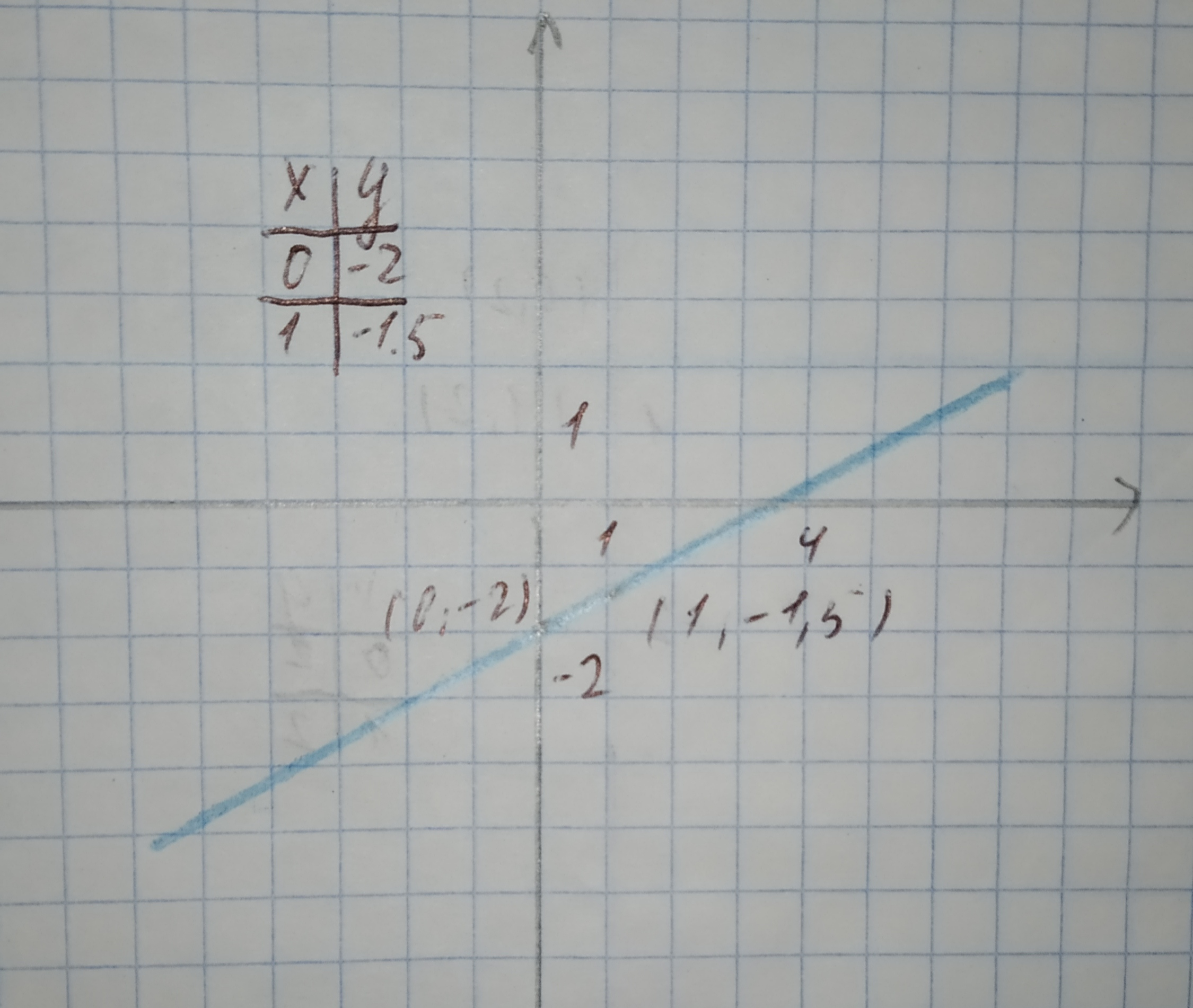Ask question

# We give linear equations. For each equation, a. find the y-intercept and slope. b. determine whether the line slopes upward, slopes downward, or is horizontal, without graphing the equation. c. use two points to graph the equation. y=0.5x−2# We give linear equations. For each equation, a. find the y-intercept and slope. b. determine whether the line slopes upward, slopes downward, or is horizontal, without graphing the equation. c. use two points to graph the equation. y=0.5x−2

Question
Linear equations and graphsasked 2020-10-31
We give linear equations. For each equation,
a. find the y-intercept and slope.
b. determine whether the line slopes upward, slopes downward, or is horizontal, without graphing the equation.
c. use two points to graph the equation.
y=0.5x−2

## Answers (1)2020-11-01
a. The y-intercept is the constant in the linear equation:
—2
The slope is the coefficient of x in the linear equation:
0.5
b. If the slope is positive, then the graph slopes upwards. If the slope is negative, then the graph slopes downwards. If the slope is 0, then the graph is horizontal.
Slope > 0 $$\displaystyle\Rightarrow$$ Slopes upwards
c. Evaluate the given equation for two values of x.
Graph the two points.
Draw a line through the two points:Results:
a.-2,0.5
b.Slopes upwards
c.See graph

### Relevant Questionsasked 2021-01-19
We give linear equations. For each equation,
a. find the y-intercept and slope.
b. determine whether the line slopes upward, slopes downward, or is horizontal, without graphing the equation.
c. use two points to graph the equation.
y=−0.75x−5asked 2020-11-17
We give linear equations. For each equation,
a. find the y-intercept and slope.
b. determine whether the line slopes upward, slopes downward, or is horizontal, without graphing the equation.
c. use two points to graph the equation.
y=−1+2xasked 2021-02-12
We give linear equations. For each equation,
a. find the y-intercept and slope.
b. determine whether the line slopes upward, slopes downward, or is horizontal, without graphing the equation.
c. use two points to graph the equation.
y=-3asked 2020-10-23
We give linear equations. For each equation,
a. find the y-intercept and slope.
b. determine whether the line slopes upward, slopes downward, or is horizontal, without graphing the equation.
c. use two points to graph the equation. y=6-7xasked 2020-11-05
Given linear equation y = -0.75x-5
a. find the y-intercept and slope.
b. determine whether the line slopes upward, slopes downward, or is horizontal, without graphing the equation.
c. use two points to graph the equation.asked 2020-11-05
Given linear equation y = 0.5x – 2
a. find the y-intercept and slope.
b. determine whether the line slopes upward, slopes downward, or is horizontal, without graphing the equation.
c. use two points to graph the equation.asked 2021-01-19
Given linear equation y = -1+2x
a. find the y-intercept and slope.
b. determine whether the line slopes upward, slopes downward, or is horizontal, without graphing the equation.
c. use two points to graph the equation.asked 2020-11-02
Given linear equation y = 3+4x
a. find the y-intercept and slope.
b. determine whether the line slopes upward, slopes downward, or is horizontal, without graphing the equation.
c. use two points to graph the equation.asked 2021-01-04
Given linear equation y = 6 − 7x
a. find the y-intercept and slope.
b. determine whether the line slopes upward, slopes downward, or is horizontal, without graphing the equation.
c. use two points to graph the equation.asked 2020-12-21
Given linear equation y = -8-4x
a. find the y-intercept and slope.
b. determine whether the line slopes upward, slopes downward, or is horizontal, without graphing the equation.
c. use two points to graph the equation.
...